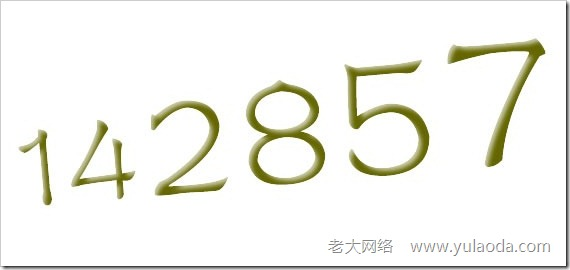142857 X 1 = 142857

142857 X 2 = 285714

142857 X 3 = 428571

142857 X 4 = 571428

142857 X 5 = 714285

142857 X 6 = 857142

142 + 857 = 999

14 + 28 + 57 = 99

20408 + 122449 = 142857

“142857”

142857×1＝142857（原数字）

142857×2＝285714（轮值）

142857×3＝428571（轮值）

142857×4＝571428（轮值）

142857×5＝714285（轮值）

142857×6＝857142（轮值）

142857×7＝999999（放假由9代班）

142857×8＝1142856（7分身，即分为头一个数字1与尾数6，数列内少了7）

142857×9＝1285713（4分身）

142857×10＝1428570（1分身）

142857×11＝1571427（8分身）

142857×12＝1714284（5分身）

142857×13＝1857141（2分身）

142857×14＝1999998（9也需要分身变大）

众数和为9的数字与任意数相乘，其结果的众数和都为9。例如306的众数和为9，而306*22=6732，数字6732的众数和也为 9（6+7+3+2=18，1+8=9）。

众数和为1的数字与任意数相乘，其结果的众数与被乘数的众数和相等。例如13的众数和为4，325的众数和为1，而325*13=4225，数字 4225的众数和也为4（4+2+2+5=13，1+3=4）。

总结得出一个普遍的规律，如果A*B=C，则众数和为A的数字与众数和为B的数字相乘，其结果的众数和亦与C的众数和相等。例如3*4=12。取一 个众数和为3的数字，如201，再取一个众数和为4的数字，如112，两数相乘，结果为201*112=22512，22512的众数和为 3（2+2+5+1+2=12，1+2=3），可见3*4=12，数字12的众数和亦为3。

另外，数字相加亦遵守此规律。例如3+4=7。求数字201和112的和，结果为313，求313的众数和，得数字7（3+1+3=7），刚好3与 4相加的结果亦为7。

4 9 2

3 5 7

8 1 6 ( 洛书)

7

2

8 3 5 4 9

1

6 (河图)

“河图”的数字图没有“洛书”数字图出名，这是因为人们未能动发现其数学规律，但是用众数和的规律去分析它，就能发现它的奇妙之处。

“河图”数字图中，任意一组数字互相进行相乘，其结果的众数和都为6。例如27165*38495=1045716675，求结果的众数 和，1+4+5+7+1+6+6+7+5=42，4+2=6，可见，结果的众数和为6。

“太极图”﹑“河图”﹑“洛书”通过种种手段暗示数字6与数字9的重要性，其中“河图”与“洛书”更是在熟悉数字众数和规律的前提下编制而成。但是，据我 们所知，数字众数和的规律刚刚被本人发现，同时也没有任何证据显示古人已经知道这数学规律。